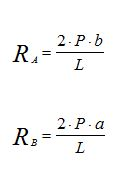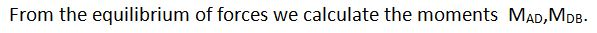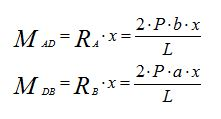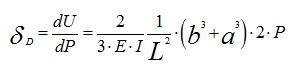Wednesday, 03 May 2017 10:44cat

Calculation Example - Calculate the deflection. Castigliano Theorem.

Written by  TheStructuralEngineer.info

Calculate the deflection at point D for the point load 2P for the pinned beam at two ends.

Solution

From the equilibrium of forces we calculate the reactions RA,RB.Strain Energy.Castigliano Theorem.